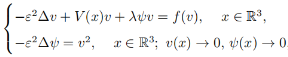# 半经典次临界增长SchrO¨dinger-Poisson方程组变号解的存在性和集中现象Existence and Concentration of Infinitely Many Sign-Changing Solutions of Semiclassical Subcritical GrowthSchrO¨dinger-Poisson Systems

DOI: 10.12677/AAM.2021.104146, PDF, 下载: 16  浏览: 47

Abstract: In this paper, we study the following semiclassical Schrödinger-Poisson system, where ε > 0 is a small parameter, λ > 0 is a parameter and V : 3 → ℝ is a bounded potential function, the nonlinearity f is superlinear at the origin and at infinity, and is subcritical growth. We proved the existence of infinitely many sign-changing solutions by the method of invariant sets with descending flow and the truncation technique, and proved that these solutions are located near the local minimum point of the potential function V as ε → 0 by the penalization method.

  Chen, Z., Qin, D. and Zhang, W. (2020) Localized Nodal Solutions of Higher Topological Type For Nonlinear Schrödinger—Poisson System. Nonlinear Analysis, 198, Article ID: 111896. https://doi.org/10.1016/j.na.2020.111896  Chen, S., Liu, J. and Wang, Z.-Q. (2019) Localized Nodal Solutions for a Critical Nonlinear Schrödinger Equations. Journal of Functional Analysis, 277, 594–640. https://doi.org/10.1016/j.jfa.2018.10.027  Chen, S. and Wang, Z.-Q. (2017) Localized Nodal Solutions of Higher Topological Type for Semiclassical Nonlinear Schrödinger Equations. Calculus of Variations and Partial Differential  Equations, 56, 1-26. https://doi.org/10.1007/s00526-016-1094-4  Liu, X., Liu, J. and Wang, Z.-Q. (2019) Localized Nodal Solutions for Quasilinear Schrödinger Equations. Journal of Differential Equations, 267, 7411-7461. https://doi.org/10.1016/j.jde.2019.08.003  Byeon, J. and Wang, Z.-Q. (2003) Standing Waves with a Critical Frequency for Nonlinear Schrödinger Equations, II. Calculus of Variations and Partial Differential Equations, 18, 207- 219. https://doi.org/10.1007/s00526-002-0191-8  Ruiz, D. (2006) The Schrödinger–Poisson Equation under the Effect of a Nonlinear Local Term.  Journal of Functional Analysis, 237, 655-674. https://doi.org/10.1016/j.jfa.2006.04.005  Cerami, G. and Vaira, G. (2010) Positive Solutions for Some Non-Autonomous Schrödinger– Poisson Systems. Journal of Differential Equations, 48, 521-543. https://doi.org/10.1016/j.jde.2009.06.017  Liu, Z. and Sun, J. (2001) Invariant Sets of Descending Flow in Critical Point Theory with Applications to Nonlinear Differential Equations. Journal of Differential Equations, 172, 257- 299. https://doi.org/10.1006/jdeq.2000.3867  Liu, J., Liu, X. and Wang, Z.-Q. (2016) Sign-Changing Solutions for Coupled Nonlinear Schrödinger Equations with Critical Growth. Journal of Differential Equations, 261, 7194- 7236. https://doi.org/10.1016/j.jde.2016.09.018  Tintarev, K. and Fieseler, K.-H. (2007) Concentration Compactness. Functional-Analytic Grounds and Applications. Imperial College Press, London.https://doi.org/10.1142/p456  Cerami, G., Devillanova, G. and Solimini, S. (2005) Infinitely Many Bound States for Some Nonlinear Scalar Field Equations. Calculus of Variations and Partial Differential Equations, 23, 139-168. https://doi.org/10.1007/s00526-004-0293-6  Devillanova, G. and Solimini, S. (2002) Concentrations Estimates and Multiple Solutions to Elliptic Problems at Critical Growth. Advances in Difference Equations, 7, 1257-1280.